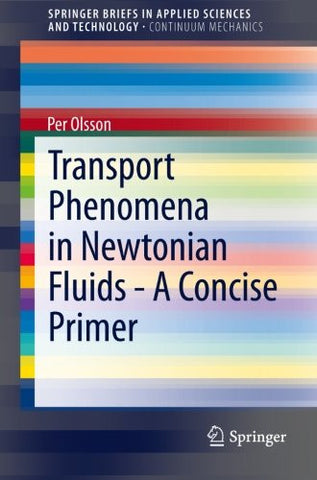Shopping Cart### Transport Phenomena in Newtonian Fluids - A Concise Primer (SpringerBriefs in Applied Sciences and Technology)

Regular price \$87.98 Sale price

Compare at \$106.67

• ISBN-13: 9783319013084
• Publisher: Springer
• Release Date: Sep 11, 2013
• Edition: 2014
• Pages: 94 pages
• Dimensions: 0.25 x 9.25 x 6.1 inches

### Overview

This short primer provides a concise and tutorial-style introduction to transport phenomena in Newtonian fluids , in particular the transport of mass, energy and momentum.

The reader will find detailed derivations of the transport equations for these phenomena, as well as selected analytical solutions to the transport equations in some simple geometries. After a brief introduction to the basic mathematics used in the text, Chapter 2, which deals with momentum transport, presents a derivation of the Navier-Stokes-Duhem equation describing the basic flow in a Newtonian fluid.  Also provided at this stage are the derivations of the Bernoulli equation, the pressure equation and the wave equation for sound waves. The boundary layer, turbulent flow and flow separation are briefly reviewed.
Chapter 3, which addresses energy transport caused by thermal conduction and convection, examines a derivation of the heat transport equation.  Finally, Chapter 4, which focuses on mass transport caused by diffusion and convection, discusses a derivation of the mass transport equation.